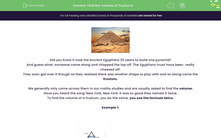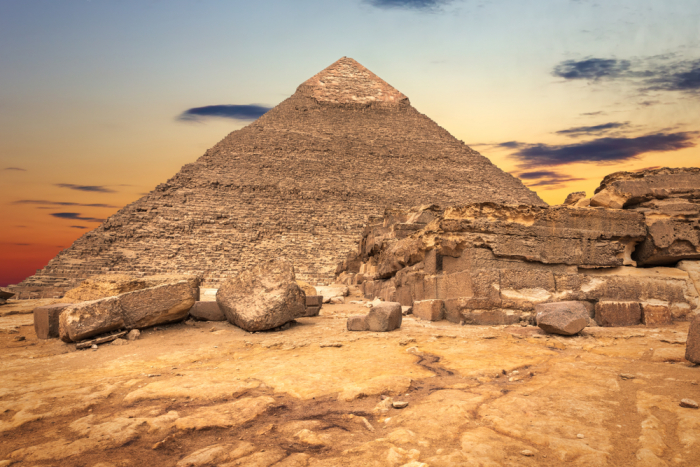# Find the Volume of Frustums

In this worksheet, students will learn how to find the volume of frustums.Key stage:  KS 4

Year:  GCSE

GCSE Subjects:   Maths

GCSE Boards:   AQA, Eduqas, Pearson Edexcel, OCR,

Curriculum topic:   Geometry and Measures, Mensuration

Curriculum subtopic:   Mensuration and Calculation Volume and Surface Area Calculations

Popular topics:   Geometry worksheets

Difficulty level:#### Worksheet OverviewDid you know it took the Ancient Egyptians 20 years to build one pyramid?

And guess what, someone came along and chopped the top off. The Egyptians must have been  really cheesed off.

They soon got over it though as they realised there was another shape to play with and so along came the frustum.

We generally only come across them in our maths studies and are usually asked to find the volume.

Have you heard the song 'New York, New York' it was so good they named it twice.

To find the volume of a frustum, you do the same, you use the formula twice.

Example 1:Find the volume of the frustum above

Recap of formula for finding the volume of a pyramid: 1/3 x base area x vertical height  - often written as 1/3Ah

Volume is measured in units³

Three simple steps:

1. Calculate the volume of the square-based pyramid.

2. Calculate the volume of the smaller pyramid as shown.

3.  Subtract the smaller volume from the larger one.

Base area of the larger pyramid: 6 x 3 = 18 cm²

Volume: 1/3 x 18 x 8 = 48 cm³

Base area of the smaller pyramid: 3 x 1 = 3 cm²

Volume: 1/3 x 3 x 3 = 3 cm³

Volume of the frustum: 48 - 3 = 45 cm³

Example 2:Find the volume of the frustum above

Recap of formula for the volume of a cone: 1/3 x π x r² x h

Base area of large cone: π  x r² = 254.47 cm² (correct to 2 decimal places)

Volume of a full cone: 1/3 x 254.47 x 9 = 763.41 cm³

Base area of the smaller cone:  π x r² = 63.62 cm² (correct to 2 decimal places)

Volume of the smaller cone: 1/3 x 63.62 x 3 = 63.62 cm³

Volume of the frustum: 763.41 - 63.62 = 699.79 cm³

In the following activities, the π button has been used on the calculator.

For 1/3 1 ÷ 3  has been used.

Round to two decimal places as you work through each part.

### What is EdPlace?

We're your National Curriculum aligned online education content provider helping each child succeed in English, maths and science from year 1 to GCSE. With an EdPlace account you’ll be able to track and measure progress, helping each child achieve their best. We build confidence and attainment by personalising each child’s learning at a level that suits them.

Get started